## Feedback

The Yankees should be winning the World Series in class examples and homeworks. Realism is essential to help fight the over-optimistic ivory tower white washing of how the world really works.

## Feedback

Please continue to provide in-person or in-survey feedback.

• Uniform
• Normal
• Exponential
• Gamma
• Beta

## Uniform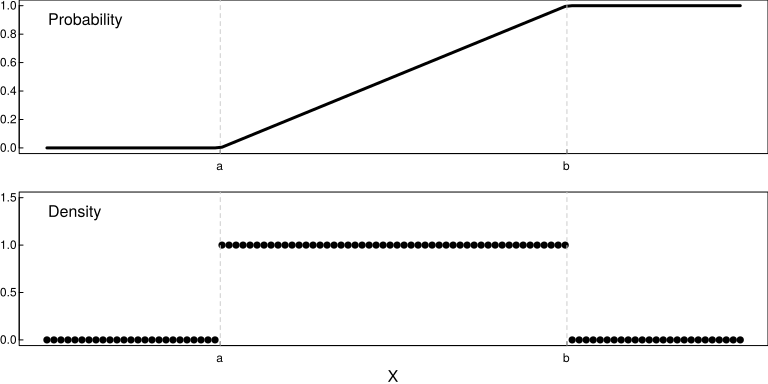## Uniform

• Two parameters:
• $$a$$, left end point
• $$b$$, right end point
• All values between $$a$$ and $$b$$ are equally likely to occur.

## Uniform

• Applications
• Generation of random variables from other distributions. (How might one generate a Bernoulli random variable from a uniform random variable?)
• Maximum uncertainty models

## Normal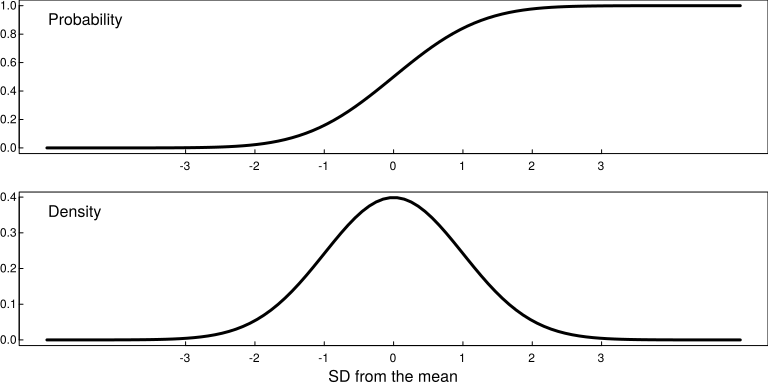## Exponential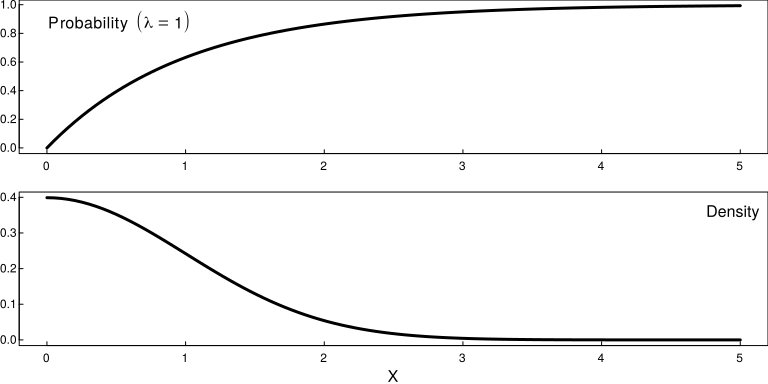## Gamma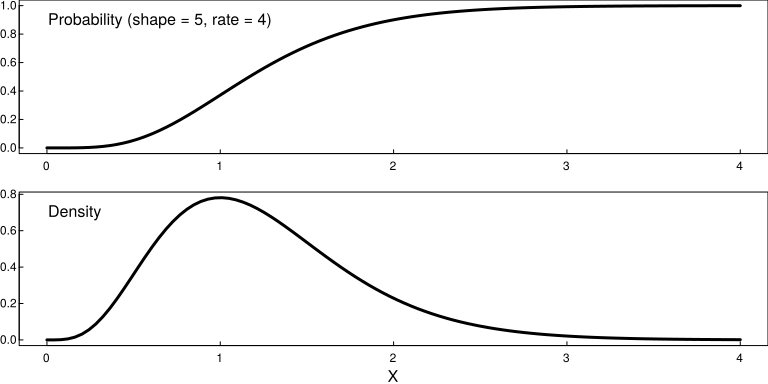## Beta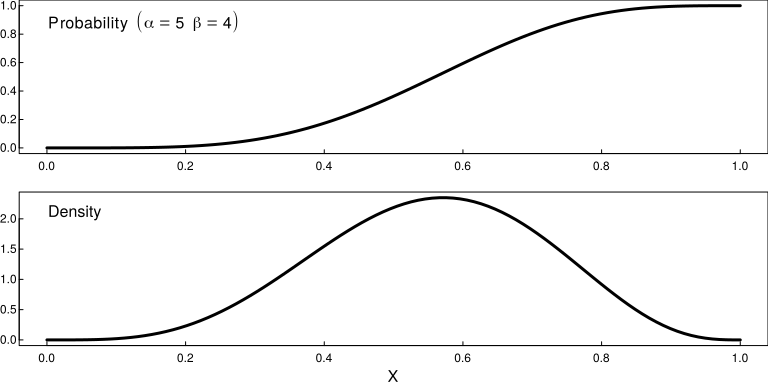## Mixture distributions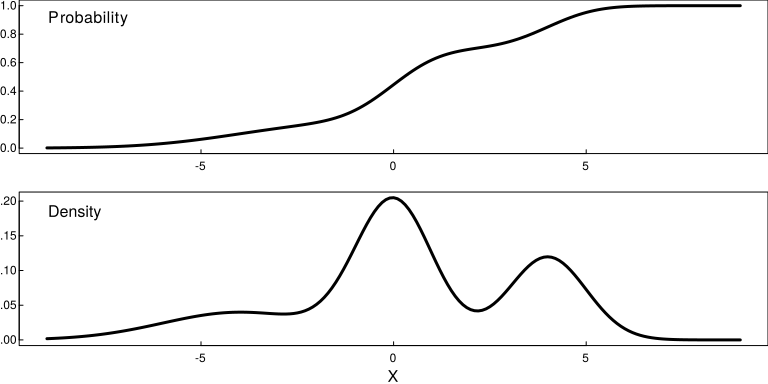## Mixture distributions (continuous and discrete)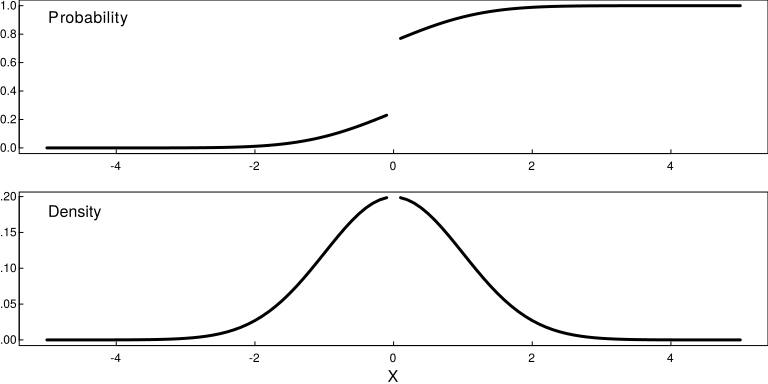## Finite Mixture Distributions

Suppose an outcome is of the following types with corresponding probability:

Type Prob
0 .25
1 .5
2 .25

## Finite Mixture Distributions

Now suppose that the distribution of outcome X depends on Type. For example,

Type Distn $$X|\text{Type}$$
0 N(0,1)
1 Exp(1)
2 Uniform(-1, 1)

## Finite Mixture Distributions

• We know the distribution of $$X|\text{Type}$$.
• What is the distribution of $$X$$?

## Finite Mixture Distributions

• We know the conditional distribution of $$X|\text{Type}$$.
• What is the marginal distribution of $$X$$?

## Finite Mixture Distributions

• Recall:
Type 0 Type 1 Type 2
$$X \leq x$$ $$P(X \leq x)$$
$$X > x$$ $$P(X > x)$$
$$p_0 = P(\text{Type } 0)$$ $$p_1 = P(\text{Type } 1)$$ $$p_2 = P(\text{Type } 2)$$ 1

## Finite Mixture Distributions

• Recall:
Type 0 Type 1 Type 2
$$X \leq x$$ $$p_0P(X\leq x|\text{Type } 0)$$ $$p_1P(X\leq x|\text{Type } 1)$$ $$p_2P(X\leq x|\text{Type } 2)$$ $$P(X \leq x)$$
$$X > x$$ $$p_0P(X > x|\text{Type } 0)$$ $$p_1P(X > x|\text{Type } 1)$$ $$p_2P(X > x|\text{Type } 2)$$ $$P(X > x)$$
$$p_0 = P(\text{Type } 0)$$ $$p_1 = P(\text{Type } 1)$$ $$p_2 = P(\text{Type } 2)$$ 1

## Finite Mixture Distributions

\begin{align*}P(X < x) = &P(\text{Type} = 0)P(X < x | \text{Type} = 0) \\ & + P(\text{Type} = 1)P(X < x | \text{Type} = 1)\\ & + P(\text{Type} = 2)P(X < x | \text{Type} = 2)\\ \end{align*}\\

## Finite Mixture Distributions

px <- function(x){
.25*pnorm(x) + .5*pexp(x) + .25*punif(x,-1,1)
}

## Finite Mixture Distributions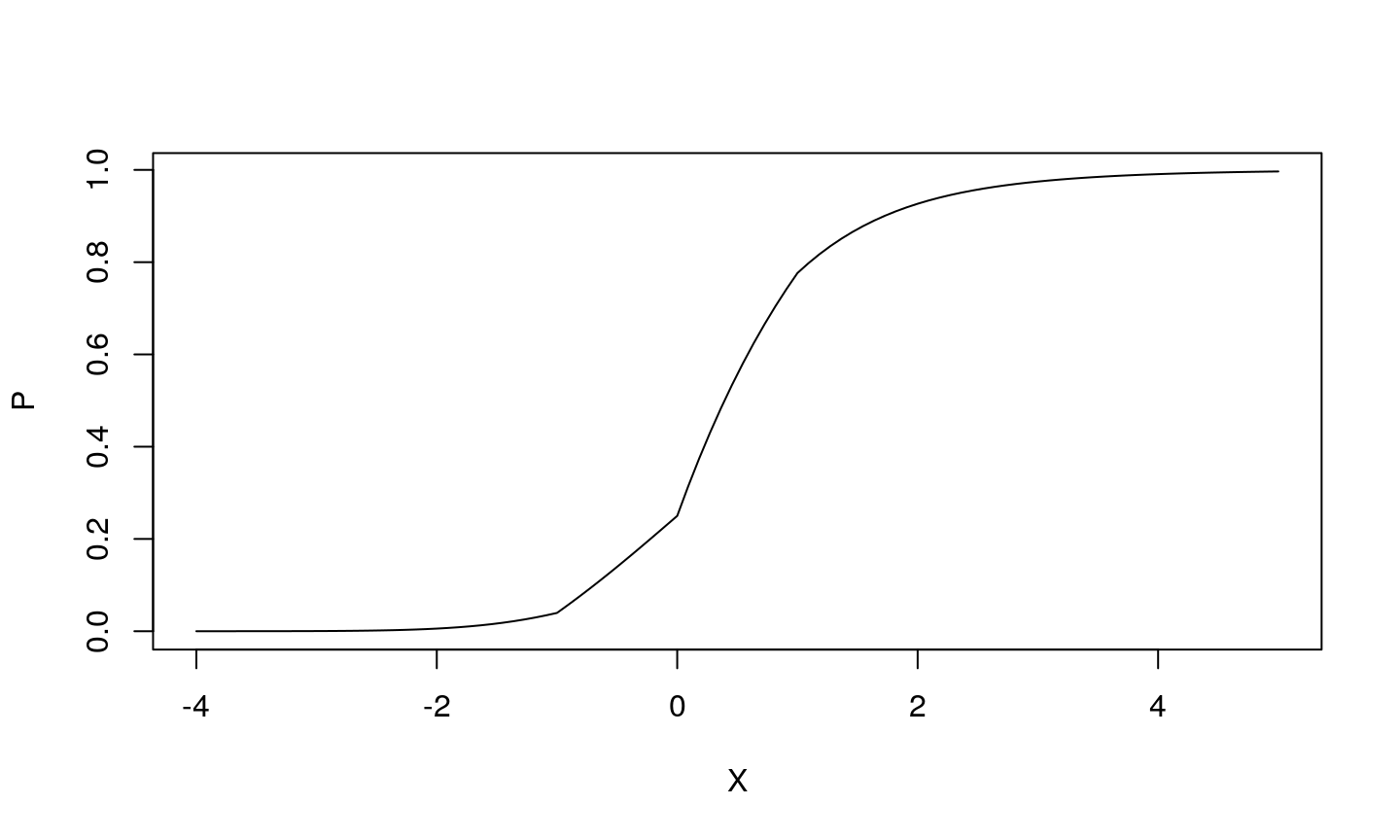## A quick note on sampling distributions

(Book section 7.2. Will come back to this.)## A quick note on sampling distributions

(Book section 7.2. Will come back to this.)